list data list nil list link list list

• Slides: 14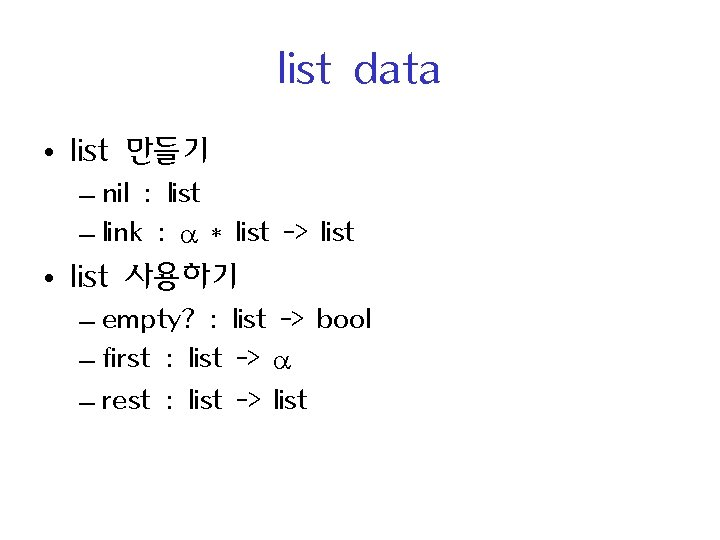list data • list 만들기 – nil : list – link : * list -> list • list 사용하기 – empty? : list -> bool – first : list -> – rest : list -> list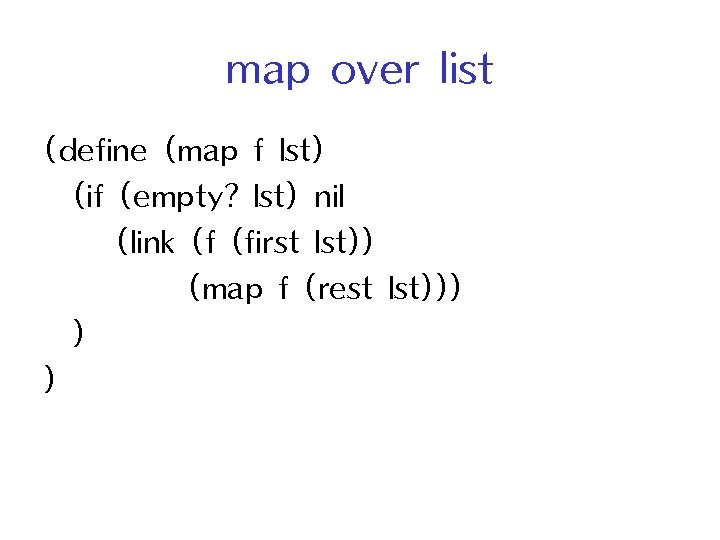map over list (define (map f lst) (if (empty? lst) nil (link (f (first lst)) (map f (rest lst))) ) )(define (inc n) (+ n 1)) (map inc ‘(1 2 3)) (define (square n) (* n n)) (map square ‘(1 2 3))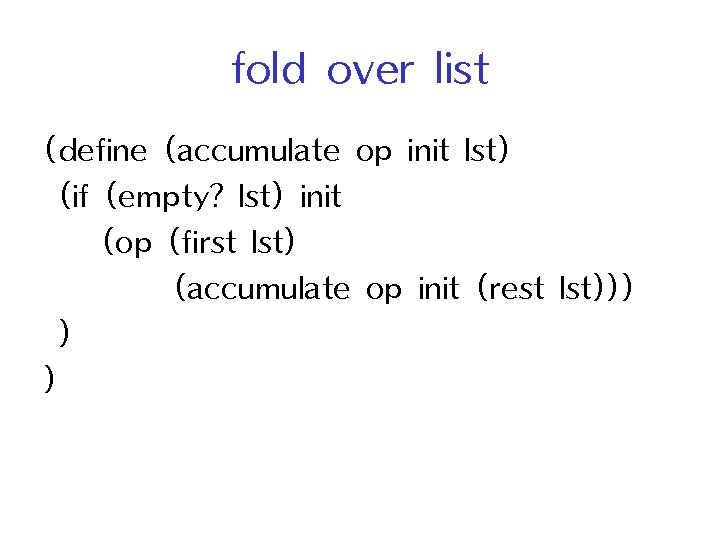fold over list (define (accumulate op init lst) (if (empty? lst) init (op (first lst) (accumulate op init (rest lst))) ) )binary tree data • tree 만들기 – leaf : -> tree – node : tree * tree -> tree • tree 사용하기 – leaf-val : tree -> – is-leaf? : tree -> bool – left-subtree : tree -> tree – right-subtree : tree -> tree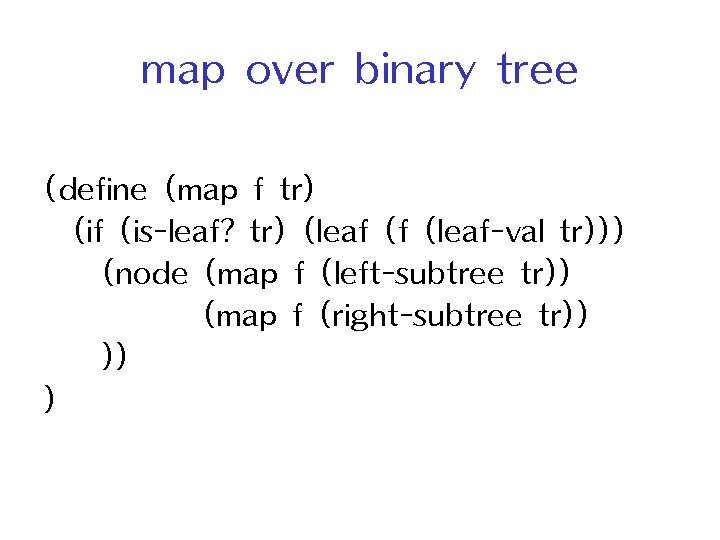map over binary tree (define (map f tr) (if (is-leaf? tr) (leaf (f (leaf-val tr))) (node (map f (left-subtree tr)) (map f (right-subtree tr)) )) )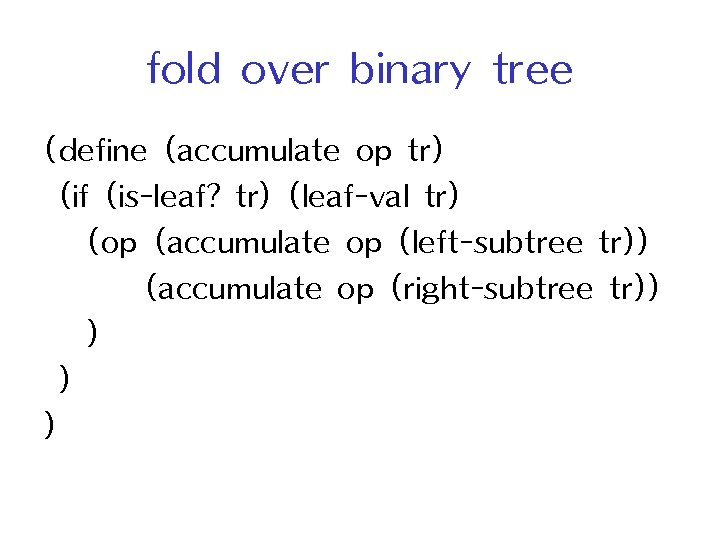fold over binary tree (define (accumulate op tr) (if (is-leaf? tr) (leaf-val tr) (op (accumulate op (left-subtree tr)) (accumulate op (right-subtree tr)) )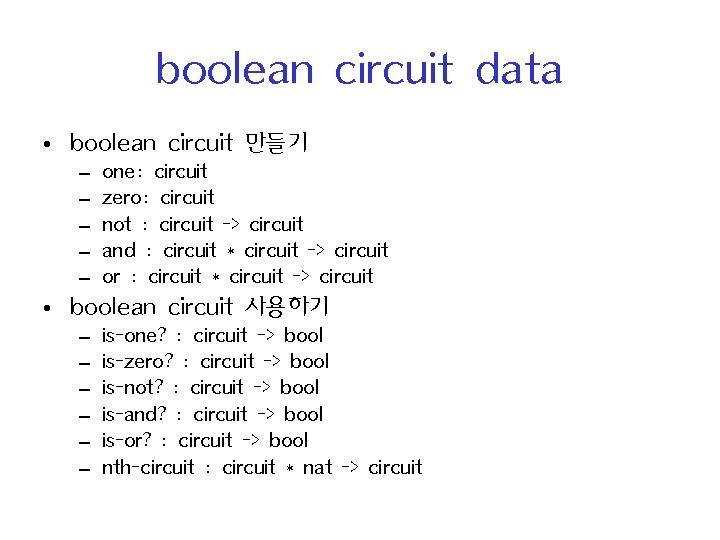boolean circuit data • boolean circuit 만들기 – – – one: circuit zero: circuit not : circuit -> circuit and : circuit * circuit -> circuit or : circuit * circuit -> circuit • boolean circuit 사용하기 – – – is-one? : circuit -> bool is-zero? : circuit -> bool is-not? : circuit -> bool is-and? : circuit -> bool is-or? : circuit -> bool nth-circuit : circuit * nat -> circuit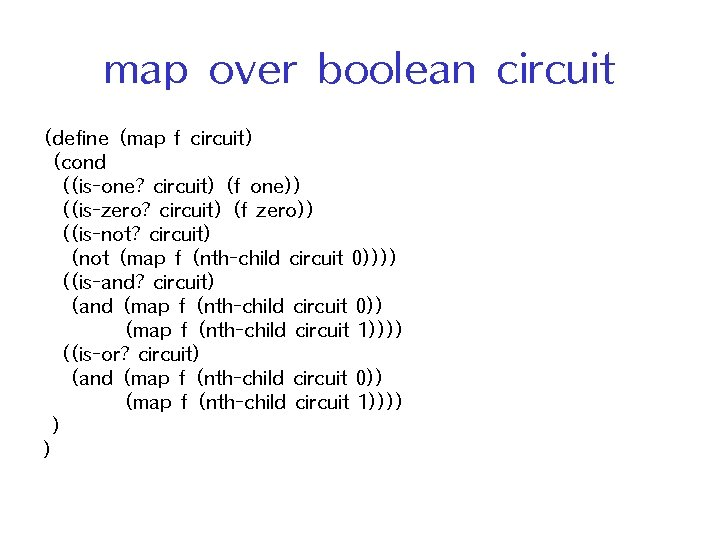map over boolean circuit (define (map f circuit) (cond ((is-one? circuit) (f one)) ((is-zero? circuit) (f zero)) ((is-not? circuit) (not (map f (nth-child circuit 0)))) ((is-and? circuit) (and (map f (nth-child circuit 0)) (map f (nth-child circuit 1)))) ((is-or? circuit) (and (map f (nth-child circuit 0)) (map f (nth-child circuit 1)))) ) )fold over boolean circuit (define (eval c) (cond ((is-one? c) 1) ((is-zero? c) 0) ((is-not? c) (bool-not (eval (nth-circuit c 0)))) ((is-and? c) (bool-and (eval (nth-circuit c 0)) (eval (nth-circuit c 1)))) ((is-or? c) (bool-or (eval (nth-circuit c 0)) (eval (nth-circuit c 1)))) ) )cascading over list (define (sum-odd lst) (accumulate + 0 (filter odd? lst) ) ) (define (sum-odd-square lst) (accumulate + 0 (map square (filter odd? lst)) ) )cascading over tree (define (sum-odd-squares tr) (accumulate + 0 (map square (filter odd? (enlist-leaves tr) ) )symbolic expression data • 식 만들기 – – const : int -> expr var : string -> expr sum : expr * expr -> expr product : expr * expr -> expr • 식 사용하기 – – – – – is-const? : expr -> bool is-var? : expr -> bool is-sum? : expr -> bool is-product? : expr -> bool const-val: expr -> int var-name: expr -> string addend: expr * nat -> expr augend: expr -> expr multiplier: expr -> expr multiplicand: expr -> expr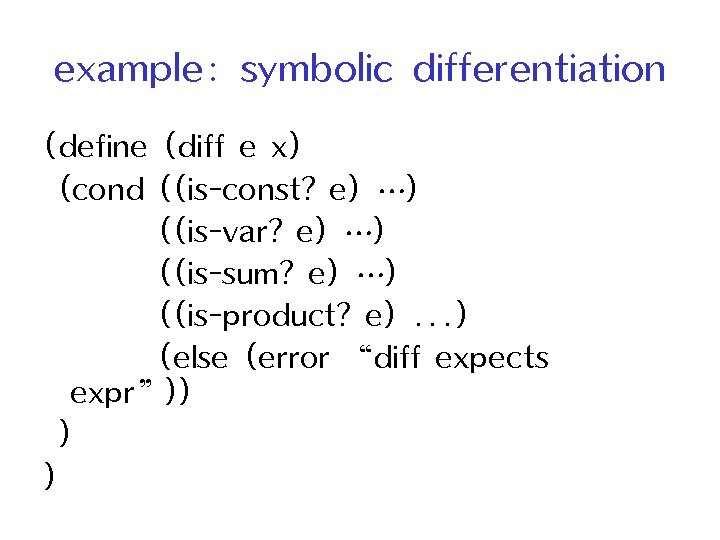example: symbolic differentiation (define (diff e x) (cond ((is-const? e) …) ((is-var? e) …) ((is-sum? e) …) ((is-product? e). . . ) (else (error “diff expects expr”)) ) )